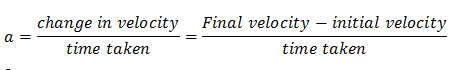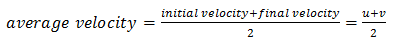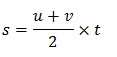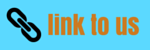# Motion

## 6. Equations of uniformly accelerated motion

• There are three equations of bodies moving with uniform acceleration which we can use to solve problems of motion

### First Equation of motion

• The first equation of motion is $v = u + at$ , where v is the final velocity and u is the initial velocity of the body.
• First equation of motion gives velocity acquired by body at any time $t$.
• Now we know that accelerationso, $a = \frac{{v - u}}{t}$
and, $at = v - u$
rearranging above equation we get first equation of motion that is
$v = u + at$

### Second Equation of motion

• Second equation of motion is
$s = ut + \frac{1}{2}a{t^2}$
where $u$ is initial velocity, $a$ is uniform acceleration and $s$ is the distance travelled by body in time $t$.
• Second equation of motion gives distance travelled by a moving body in time $t$.
• To obtain second equation of motion consider a body with initial velocity $u$ moving with acceleration a for time $t$ its final velocity at this time be $v$. If body covered distance $s$ in this time $t$ , then average velocity of the body would beDistance travelled by the body isFrom first equation of motion
$v = u + at$
So putting first equation of motion in above equation we get ,
$s = \frac{{u + u + at}}{2} \times t = \frac{{\left( {2u + at} \right)t}}{2} = \frac{{2ut + a{t^2}}}{2}$
Rearranging it we get
$s = ut + \frac{1}{2}a{t^2}$

### Third equation of motion

• Third equation of motion is
${v^2} = {u^2} + 2as$ where $u$ is initial velocity, $v$ is the final velocity, $a$ is uniform acceleration and $s$ is the distance travelled by the body.
• This equation gives the velocity acquired by the body in travelling a distance $s$.
• Third equation of motion can be obtained by eliminating time t between first and second equations of motion.
So, first and second equations of motion respectively are
$v = u + at$ and $s = ut + \frac{1}{2}a{t^2}$
Rearranging first equation of motion to find time t we get
$t = \frac{{v - u}}{a}$
Putting this value of t in second equation of motion we get
$s = \frac{{u\left( {v - u} \right)}}{a} + \frac{1}{2}a{\left( {\frac{{v - u}}{a}} \right)^2}$

$s = \frac{{uv - {u^2}}}{a} + \frac{{a\left( {{v^2} + {u^2} - 2uv} \right)}}{{2{a^2}}}$

$s = \frac{{2uv - 2{u^2} + {v^2} + {u^2} - 2uv}}{{2a}}$

Rearranging it we get
${v^2} = {u^2} + 2as$
• These three equations of motion are used to solve uniformly accelerated motion problems and following three important points should be remembered while solving problems
1. if a body starts moving from rest its initial velocity $u = 0$
2. if a body comes to rest i.e., it stops then its final velocity would be $v = 0$
3. If a body moves with uniform velocity then its acceleration would be zero.

## Test your Knowlegde

1)The __________________ equation of motion is v=u+at , where v is the final velocity and u is the initial velocity of the body.
2)Second equation of motion is  s=ut+1/2at2  where u is initial velocity, a is uniform acceleration and s is the ____________
______ travelled by body in time t.
3) Third equation of motion is v2=u2+2as where u is initial velocity, v is the final velocity, a is __________ and s is the distance travelled by the body.